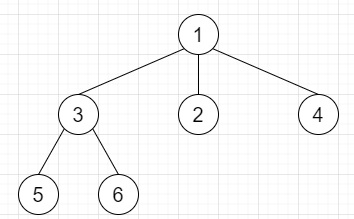# Serialize and Deserialize N-ary Tree in C++

Suppose we have one N-ary tree and we have to serialize and deserialize them. As we know that the serialization is the process of converting a data structure or object into a sequence of bits so we can store them in a file or memory buffer, and that can be reconstructed later in the same or another computer environment.

Here we have to devise an algorithm to serialize and deserialize an N-ary tree. The N-ary tree is a rooted tree in which each node has no more than N children.

So, if the input is likethen the output will be Serialize: 1 #3 2 4 #5 6 ##### and Deserialized Tree: 1[3[5, 6, ], 2, 4, ]

To solve this, we will follow these steps −

• Define a function createVector(), this will take s,

• Define one 2D array ret

• Define an array tempv

• temp := empty string

• for initialize i := 0, when i < size of s, update (increase i by 1), do −

• if s[i] is not equal to blank space and s[i] is not equal to '#', then −

• temp := temp + s[i]

• otherwise when s[i] is same as blank string, then −

• insert temp s integer at the end of tempv

• temp := empty string

• otherwise when s[i] is same as '#', then −

• insert tempv at the end of ret

• temp := empty string

• clear tempv

• while (not ret is empty and last element of ret is same as 0), do −

• delete last element from ret

• return ret

• Define a function serialize(), this will take root,

• ret := empty string

• if not root is non-zero, then −

• return ret

• Define one queue q

• insert root into q

• rret := ret concatenate val of root

• ret := ret concatenate space

• ret := ret concatenate "#"

• while (not q is empty), do −

• curr = first element of q

• delete element from q

• for initialize i := 0, when i < size of the children array of curr, update (increase i by 1), do −

• if children[i] of curr, then −

• ret := ret concatenate children[i] of curr

• insert children[i] of curr into q

• ret := ret + blank string

• ret := ret concatenate "#"

• return ret

• Define a function deserialize(), this will take data,

• if size of data is same as 0, then −

• return null

• Define one 2D array v := createVector(data)

• ret := new node with value v[0, 0]

• Define one queue q

• insert ret into q

• i := 1

• while (not q is empty and i − size of v), do −

• curr = first element of q

• delete element from q

• for initialize j := 0, when j − size of v[i],update (increase j by 1), do −

• node := v[i, j]

• temp = new node with value node

• insert temp at the end of children of curr

• insert temp into q

• (increase i by 1)

• return ret

## Example

Let us see the following implementation to get better understanding −

Live Demo

#include <bits/stdc++.h>
using namespace std;
class Node {
public:
int val;
vector<Node*> children;
Node() {}
Node(int _val) {
val = _val;
}
Node(int _val, vector<Node*> _children) {
val = _val;
children = _children;
}
};
string n_ary_to_str(Node *root){
string ret = "";
if(root){
ret = ret + to_string(root->val);
if(root->children.size() > 0){
ret += "[";
for(Node* child : root->children){
ret += n_ary_to_str(child) + ", ";
}
ret += "]";
}
}
return ret;
}
class Codec {
public:
vector<vector<int>>createVector(string s) {
vector<vector<int>> ret;
vector<int> tempv;
string temp = "";
for (int i = 0; i < s.size(); i++) {
if (s[i] != ' ' && s[i] != '#') {
temp += s[i];
}
else if (s[i] == ' ') {
tempv.push_back(stoi(temp));
temp = "";
}
else if (s[i] == '#') {
ret.push_back(tempv);
temp = "";
tempv.clear();
}
}
while (!ret.empty() && ret.back().size() == 0)
ret.pop_back();
return ret;
}
string serialize(Node *root) {
string ret = "";
if (!root)
return ret;
queue<Node *> q;
q.push(root);
ret += to_string(root->val);
ret += " ";
ret += "#";
while (!q.empty()) {
Node *curr = q.front();
q.pop();
for (int i = 0; i < curr->children.size(); i++) {
if (curr->children[i]) {
ret += to_string(curr->children[i]->val);
q.push(curr->children[i]);
}
ret += " ";
}
ret += "#";
}
return ret;
}
Node *deserialize(string data) {
Node *ret;
if (data.size() == 0)
return NULL;
vector<vector<int>> v = createVector(data);
ret = new Node(v);
queue<Node *> q;
q.push(ret);
int i = 1;
while (!q.empty() && i < v.size()) {
Node *curr = q.front();
q.pop();
for (int j = 0; j < v[i].size(); j++) {
int node = v[i][j];
Node *temp = new Node(node);
curr->children.push_back(temp);
q.push(temp);
}
i++;
}
return ret;
}
};
main() {
Codec ob;
Node n5(5), n6(6);
Node n3(3); n3.children.push_back(&n5); n3.children.push_back(&n6);
Node n2(2), n4(4);
Node n1(1); n1.children.push_back(&n3); n1.children.push_back(&n2);
n1.children.push_back(&n4);
cout << "Given Tree: " << n_ary_to_str(&n1) << endl;
string ser = ob.serialize(&n1);
cout << "Serialize: " << ser << endl;
Node *deser = ob.deserialize(ser);
cout << "Deserialized Tree: " << n_ary_to_str(deser);
}

## Input

Node n5(5), n6(6);
Node n3(3); n3.children.push_back(&n5); n3.children.push_back(&n6);
Node n2(2), n4(4);
Node n1(1); n1.children.push_back(&n3); n1.children.push_back(&n2);
n1.children.push_back(&n4);

## Output

Given Tree: 1[3[5, 6, ], 2, 4, ]
Serialize: 1 #3 2 4 #5 6 #####
Deserialized Tree: 1[3[5, 6, ], 2, 4, ]